Skip to main content
$$\newcommand{\id}{\mathrm{id}}$$ $$\newcommand{\Span}{\mathrm{span}}$$ $$\newcommand{\kernel}{\mathrm{null}\,}$$ $$\newcommand{\range}{\mathrm{range}\,}$$ $$\newcommand{\RealPart}{\mathrm{Re}}$$ $$\newcommand{\ImaginaryPart}{\mathrm{Im}}$$ $$\newcommand{\Argument}{\mathrm{Arg}}$$ $$\newcommand{\norm}{\| #1 \|}$$ $$\newcommand{\inner}{\langle #1, #2 \rangle}$$ $$\newcommand{\Span}{\mathrm{span}}$$

# 11.E: Parametric Equations and Polar Coordinates (Exercises)

$$\newcommand{\vecs}{\overset { \rightharpoonup} {\mathbf{#1}} }$$

$$\newcommand{\vecd}{\overset{-\!-\!\rightharpoonup}{\vphantom{a}\smash {#1}}}$$

## 11.1: Parametric Equations

For the following exercises, sketch the curves below by eliminating the parameter t. Give the orientation of the curve.

1) $$\displaystyle x=t^2+2t, y=t+1$$

Solution: orientation: bottom to top2) $$\displaystyle x=cos(t),y=sin(t),(0,2π]$$

3) $$\displaystyle x=2t+4,y=t−1$$

Solution: orientation: left to right4) $$\displaystyle x=3−t,y=2t−3,1.5≤t≤3$$

For the following exercises, eliminate the parameter and sketch the graphs.

5) $$\displaystyle x=2t^2,y=t^4+1$$

Solution: $$\displaystyle y=\frac{x^2}{4}+1$$For the following exercises, use technology (CAS or calculator) to sketch the parametric equations.

6) [T] $$\displaystyle x=t^2+t,y=t^2−1$$

7) [T] $$\displaystyle x=e^{−t},y=e^{2t}−1$$

Solution: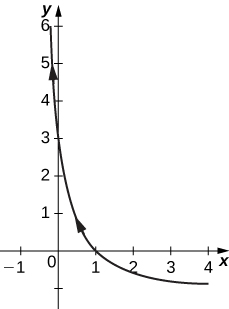8) [T] $$\displaystyle x=3cost,y=4sint$$

9) [T] $$\displaystyle x=sect,y=cost$$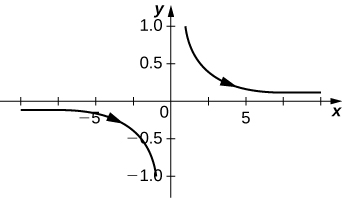For the following exercises, sketch the parametric equations by eliminating the parameter. Indicate any asymptotes of the graph.

10) $$\displaystyle x=e^t,y=e^{2t}+1$$

11) $$\displaystyle x=6sin(2θ),y=4cos(2θ)$$

Solution:12) $$\displaystyle x=cosθ,y=2sin(2θ)$$

13) $$\displaystyle x=3−2cosθ,y=−5+3sinθ$$

Solution: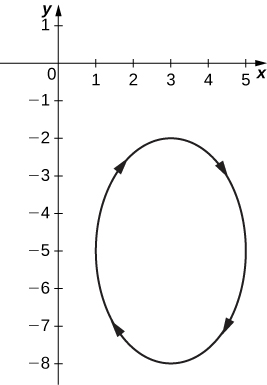14) $$\displaystyle x=4+2cosθ,y=−1+sinθ$$

15) $$\displaystyle x=sect,y=tant$$

Solution: Asymptotes are $$\displaystyle y=x$$ and $$\displaystyle y=−x$$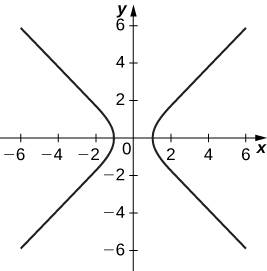16) $$\displaystyle x=ln(2t),y=t^2$$

17) $$\displaystyle x=e^t,y=e^{2t}$$

Solution:18) $$\displaystyle x=e^{−2t},y=e^{3t}$$

19) $$\displaystyle x=t^3,y=3lnt$$

Solution:20) $$\displaystyle x=4secθ,y=3tanθ$$

For the following exercises, convert the parametric equations of a curve into rectangular form. No sketch is necessary. State the domain of the rectangular form.

21) $$\displaystyle x=t^2−1,y=\frac{t}{2}$$

Solution: $$\displaystyle x=4y^2−1;$$ domain: $$\displaystyle x∈[1,∞)$$.

22) $$\displaystyle x=\frac{1}{\sqrt{t+1}},y=\frac{t}{1+t},t>−1$$

23) $$\displaystyle x=4cosθ,y=3sinθ,t∈(0,2π]$$

Solution: $$\displaystyle \frac{x^2}{16}+\frac{y^2}{9}=1;$$ domain $$\displaystyle x∈[−4,4].$$

24) $$\displaystyle x=cosht,y=sinht$$

25) $$\displaystyle x=2t−3,y=6t−7$$

Solution: $$\displaystyle y=3x+2;$$ domain: all real numbers.

26) $$\displaystyle x=t^2,y=t^3$$

27) $$\displaystyle x=1+cost,y=3−sint$$

Solution: $$\displaystyle (x−1)^2+(y−3)^2=1$$; domain: $$\displaystyle x∈[0,2]$$.

28) $$\displaystyle x=\sqrt{t},y=2t+4$$

29) $$\displaystyle x=sect,y=tant,π≤t<\frac{3π}{2}$$

Solution: $$\displaystyle y=\sqrt{x^2−1}$$; domain: $$\displaystyle x∈[−1,1]$$.

30) $$\displaystyle x=2cosht,y=4sinht$$

31) $$\displaystyle x=cos(2t),y=sint$$

Solution: $$\displaystyle y^2=\frac{1−x}{2};$$ domain: $$\displaystyle x∈[2,∞)∪(−∞,−2].$$

32) $$\displaystyle x=4t+3,y=16t^2−9$$

33) $$\displaystyle x=t^2,y=2lnt,t≥1$$

Solution: $$\displaystyle y=lnx;$$ domain: $$\displaystyle x∈(0,∞).$$

34) $$\displaystyle x=t3^,y=3lnt,t≥1$$

35) $$\displaystyle x=t^n,y=nlnt,t≥1,$$ where n is a natural number

Solution: $$\displaystyle y=lnx;$$ domain: $$\displaystyle x∈(0,∞).$$

36) $$\displaystyle x=ln(5t)$$ $$\displaystyle y=ln(t^2)$$ where $$\displaystyle 1≤t≤e$$

37) $$\displaystyle x=2sin(8t)$$ $$\displaystyle y=2cos(8t)$$

Solution: $$\displaystyle x^2+y^2=4;$$ domain: $$\displaystyle x∈[−2,2].$$

38) $$\displaystyle x=tant$$ $$\displaystyle y=sec^2t−1$$

For the following exercises, the pairs of parametric equations represent lines, parabolas, circles, ellipses, or hyperbolas. Name the type of basic curve that each pair of equations represents.

39) $$\displaystyle x=3t+4$$ $$\displaystyle y=5t−2$$

Solutin: line

40) $$\displaystyle x−4=5t$$ $$\displaystyle y+2=t$$

41) $$\displaystyle x=2t+1$$ $$\displaystyle y=t^2−3$$

Solution: parabola

42) $$\displaystyle x=3cost$$ $$\displaystyle y=3sint$$

43) $$\displaystyle x=2cos(3t)$$ $$\displaystyle y=2sin(3t)$$

Solution: circle

44) $$\displaystyle x=cosht$$ $$\displaystyle y=sinht$$

45) $$\displaystyle x=3cost$$ $$\displaystyle y=4sint$$

Solutin: ellipse

46) $$\displaystyle x=2cos(3t)$$ $$\displaystyle y=5sin(3t)$$

47) $$\displaystyle x=3cosh(4t)$$ $$\displaystyle y=4sinh(4t)$$

Solution: hyperbola

48) $$\displaystyle x=2cosht$$ $$\displaystyle y=2sinht$$

49) Show that $$\displaystyle x=h+rcosθ$$ $$\displaystyle y=k+rsinθ$$ represents the equation of a circle.

50) Use the equations in the preceding problem to find a set of parametric equations for a circle whose radius is 5 and whose center is $$\displaystyle (−2,3)$$.

For the following exercises, use a graphing utility to graph the curve represented by the parametric equations and identify the curve from its equation.

51) [T] $$\displaystyle x=θ+sinθ$$ $$\displaystyle y=1−cosθ$$

Solution: The equations represent a cycloid.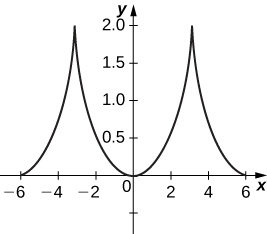52) [T] $$\displaystyle x=2t−2sint$$ $$\displaystyle y=2−2cost$$

53) [T] $$\displaystyle x=t−0.5sint$$ $$\displaystyle y=1−1.5cost$$

Solution:54) An airplane traveling horizontally at 100 m/s over flat ground at an elevation of 4000 meters must drop an emergency package on a target on the ground. The trajectory of the package is given by $$\displaystyle x=100t,y=−4.9t^2+4000,t≥0$$ where the origin is the point on the ground directly beneath the plane at the moment of release. How many horizontal meters before the target should the package be released in order to hit the target?

55) The trajectory of a bullet is given by $$\displaystyle x=v_0(cosα)ty=v_0(sinα)t−\frac{1}{2}gt^2$$ where $$\displaystyle v_0=500m/s, g=9.8=9.8m/s^2$$, and $$\displaystyle α=30degrees.$$ When will the bullet hit the ground? How far from the gun will the bullet hit the ground?

Solution: 22,092 meters at approximately 51 seconds.

56) [T] Use technology to sketch the curve represented by $$\displaystyle x=sin(4t),y=sin(3t),0≤t≤2π$$.

57) [T] Use technology to sketch $$\displaystyle x=2tan(t),y=3sec(t),−π<t<π.$$

Solution:58) Sketch the curve known as an epitrochoid, which gives the path of a point on a circle of radius b as it rolls on the outside of a circle of radius a. The equations are

$$\displaystyle x=(a+b)cost−c⋅cos[\frac{(a+b)t}{b}]$$ $$\displaystyle y=(a+b)sint−c⋅sin[\frac{(a+b)t}{b}]$$.

Let $$\displaystyle a=1,b=2,c=1. 59) [T] Use technology to sketch the spiral curve given by \(\displaystyle x=tcos(t),y=tsin(t)$$ from $$\displaystyle −2π≤t≤2π.$$60) [T] Use technology to graph the curve given by the parametric equations $$\displaystyle x=2cot(t),y=1−cos(2t),−π/2≤t≤π/2.$$ This curve is known as the witch of Agnesi.

61) [T] Sketch the curve given by parametric equations $$\displaystyle x=cosh(t)$$ $$\displaystyle y=sinh(t),$$ where $$\displaystyle −2≤t≤2.$$

Solution:## 11.2: Calculus of Parametric Curves

For the following exercises, each set of parametric equations represents a line. Without eliminating the parameter, find the slope of each line.

1) $$\displaystyle x=3+t,y=1−t$$

2) $$\displaystyle x=8+2t,y=1$$

Solution: 0

3) $$\displaystyle x=4−3t,y=−2+6t$$

4) $$\displaystyle x=−5t+7,y=3t−1$$

Solution: $$\displaystyle \frac{−3}{5}$$

For the following exercises, determine the slope of the tangent line, then find the equation of the tangent line at the given value of the parameter.

5) $$\displaystyle x=3sint,y=3cost,t=\frac{π}{4}$$

6) $$\displaystyle x=cost,y=8sint,t=\frac{π}{2}$$

Solution: $$\displaystyle Slope=0; y=8.$$

7) $$\displaystyle x=2t,y=t^3,t=−1$$

8) $$\displaystyle x=t+\frac{1}{t},y=t−\frac{1}{t},t=1$$

Solution: Slope is undefined; $$\displaystyle x=2$$.

9) $$\displaystyle x=\sqrt{t},y=2t,t=4$$

For the following exercises, find all points on the curve that have the given slope.

10) $$\displaystyle x=4cost,y=4sint,$$ slope = 0.5

Solution: $$\displaystyle t=arctan(−2); (\frac{4}{\sqrt{5}},\frac{−8}{\sqrt{5}})$$.

11) $$\displaystyle x=2cost,y=8sint,slope=−1$$

12) $$\displaystyle x=t+\frac{1}{t},y=t−\frac{1}{t},slope=1$$

Solution: No points possible; undefined expression.

13) $$\displaystyle x=2+\sqrt{t},y=2−4t,slope=0$$

For the following exercises, write the equation of the tangent line in Cartesian coordinates for the given parameter t.

14) $$\displaystyle x=e^{\sqrt{t}},y=1−lnt^2,t=1$$

Solution: $$\displaystyle y=−(\frac{2}{e})x+3$$

15) $$\displaystyle x=tlnt,y=sin^2t,t=\frac{π}{4}$$

16) $$\displaystyle x=e^t,y=(t−1)^2,at(1,1)$$

Solution: $$\displaystyle y=2x−7$$

17) For $$\displaystyle x=sin(2t),y=2sint$$ where $$\displaystyle 0≤t<2π.$$ Find all values of t at which a horizontal tangent line exists.

18) For $$\displaystyle x=sin(2t),y=2sint$$ where $$\displaystyle 0≤t<2π$$. Find all values of t at which a vertical tangent line exists.

Solution: $$\displaystyle \frac{π}{4},\frac{5π}{4},\frac{3π}{4},\frac{7π}{4}$$

19) Find all points on the curve $$\displaystyle x=4cos(t),y=4sin(t)$$ that have the slope of $$\displaystyle \frac{1}{2}$$.

20) Find $$\displaystyle \frac{dy}{dx}$$ for $$\displaystyle x=sin(t),y=cos(t)$$.

Solution: $$\displaystyle \frac{dy}{dx}=−tan(t)$$

21) Find the equation of the tangent line to $$\displaystyle x=sin(t),y=cos(t)$$ at $$\displaystyle t=\frac{π}{4}$$.

22) For the curve $$\displaystyle x=4t,y=3t−2,$$ find the slope and concavity of the curve at $$\displaystyle t=3$$.

Solution: $$\displaystyle \frac{dy}{dx}=\frac{3}{4}$$ and $$\displaystyle \frac{d^2y}{dx^2}=0$$, so the curve is neither concave up nor concave down at $$\displaystyle t=3$$. Therefore the graph is linear and has a constant slope but no concavity.

23) For the parametric curve whose equation is $$\displaystyle x=4cosθ,y=4sinθ$$, find the slope and concavity of the curve at $$\displaystyle θ=\frac{π}{4}$$.

24) Find the slope and concavity for the curve whose equation is $$\displaystyle x=2+secθ,y=1+2tanθ$$ at $$\displaystyle θ=\frac{π}{6}$$.

Solution: $$\displaystyle \frac{dy}{dx}=4,\frac{d^2y}{dx^2}=−6\sqrt{3};$$ the curve is concave down at $$\displaystyle θ=\frac{π}{6}$$.

25) Find all points on the curve $$\displaystyle x=t+4,y=t^3−3t$$ at which there are vertical and horizontal tangents.

26) Find all points on the curve $$\displaystyle x=secθ,y=tanθ$$ at which horizontal and vertical tangents exist.

Solution: No horizontal tangents. Vertical tangents at $$\displaystyle (1,0),(−1,0)$$.

For the following exercises, find $$\displaystyle d^2y/dx^2$$.

27) $$\displaystyle x=t^4−1,y=t−t^2$$

28) $$\displaystyle x=sin(πt),y=cos(πt)$$

Solution: $$\displaystyle −sec^3(πt)$$

29) $$\displaystyle x=e^{−t},y=te^{2t}$$

For the following exercises, find points on the curve at which tangent line is horizontal or vertical.

30) $$\displaystyle x=t(t^2−3),y=3(t^2−3)$$

Solution: Horizontal $$\displaystyle (0,−9)$$; vertical $$\displaystyle (±2,−6).$$

31) $$\displaystyle x=\frac{3t}{1+t^3},y=\frac{3t^2}{1+t^3}$$

For the following exercises, find $$\displaystyle dy/dx$$ at the value of the parameter.

32) $$\displaystyle x=cost,y=sint,t=\frac{3π}{4}$$

Solution: 1

33) $$\displaystyle x=\sqrt{t},y=2t+4,t=9$$

34) $$\displaystyle x=4cos(2πs),y=3sin(2πs),s=−\frac{1}{4}$$

Solution: 0

For the following exercises, find $$\displaystyle d^2y/dx^2$$ at the given point without eliminating the parameter.

35) $$\displaystyle x=\frac{1}{2}t^2,y=\frac{1}{3}t^3,t=2$$

36) $$\displaystyle x=\sqrt{t},y=2t+4,t=1$$

Solution: 4

37) Find t intervals on which the curve $$\displaystyle x=3t^2,y=t^3−t$$ is concave up as well as concave down.

38) Determine the concavity of the curve $$\displaystyle x=2t+lnt,y=2t−lnt$$.

Solution: Concave up on $$\displaystyle t>0$$.

39) Sketch and find the area under one arch of the cycloid $$\displaystyle x=r(θ−sinθ),y=r(1−cosθ)$$.

40) Find the area bounded by the curve $$\displaystyle x=cost,y=e^t,0≤t≤\frac{π}{2}$$ and the lines $$\displaystyle y=1$$ and $$\displaystyle x=0$$.

Solution: 1

41) Find the area enclosed by the ellipse $$\displaystyle x=acosθ,y=bsinθ,0≤θ<2π.$$

42) Find the area of the region bounded by $$\displaystyle x=2sin^2θ,y=2sin^2θtanθ$$, for $$\displaystyle 0≤θ≤\frac{π}{2}$$.

Solution: $$\displaystyle \frac{3π}{2}$$

For the following exercises, find the area of the regions bounded by the parametric curves and the indicated values of the parameter.

43) $$\displaystyle x=2cotθ,y=2sin^2θ,0≤θ≤π$$

44) [T] $$\displaystyle x=2acost−acos(2t),y=2asint−asin(2t),0≤t<2π$$

Solution: $$\displaystyle 6πa^2$$

45) [T] $$\displaystyle x=asin(2t),y=bsin(t),0≤t<2π$$ (the “hourglass”)

46) [T] $$\displaystyle x=2acost−asin(2t),y=bsint,0≤t<2π$$ (the “teardrop”)

Solution: $$\displaystyle 2πab$$

For the following exercises, find the arc length of the curve on the indicated interval of the parameter.

47) $$\displaystyle x=4t+3,y=3t−2,0≤t≤2$$

48) $$\displaystyle x=\frac{1}{3}t^3,y=\frac{1}{2}t^2,0≤t≤1$$

Soluton: $$\displaystyle \frac{1}{3}(2\sqrt{2}−1)$$

49) $$\displaystyle x=cos(2t),y=sin(2t),0≤t≤\frac{π}{2}$$

50) $$\displaystyle x=1+t^2,y=(1+t)^3,0≤t≤1$$

Solution: 7.075

51) $$\displaystyle x=e^tcost,y=e^tsint,0≤t≤\frac{π}{2}$$ (express answer as a decimal rounded to three places)

52) $$\displaystyle x=acos^3θ,y=asin^3θ$$ on the interval $$\displaystyle [0,2π)$$ (the hypocycloid)

Solution: $$\displaystyle 6a$$

53) Find the length of one arch of the cycloid $$\displaystyle x=4(t−sint),y=4(1−cost).$$

54) Find the distance traveled by a particle with position $$\displaystyle (x,y)$$ as t varies in the given time interval: $$\displaystyle x=sin^2t,y=cos^2t,0≤t≤3π$$.

Solution: $$\displaystyle 6\sqrt{2}$$

55) Find the length of one arch of the cycloid $$\displaystyle x=θ−sinθ,y=1−cosθ$$.

56) Show that the total length of the ellipse $$\displaystyle x=4sinθ,y=3cosθ$$ is $$\displaystyle L=16∫^{π/2}_0\sqrt{1−e^2sin^2θ}dθ$$, where $$\displaystyle e=\frac{c}{a}$$ and $$\displaystyle c=\sqrt{a^2−b^2}$$.

57) Find the length of the curve $$\displaystyle x=e^t−t,y=4e^{t/2},−8≤t≤3.$$

For the following exercises, find the area of the surface obtained by rotating the given curve about the x-axis.

58) $$\displaystyle x=t^3,y=t^2,0≤t≤1$$

Solution: $$\displaystyle \frac{2π(247\sqrt{13}+64)}{1215}$$

59) $$\displaystyle x=acos^3θ,y=asin^3θ,0≤θ≤\frac{π}{2}$$

60) [T] Use a CAS to find the area of the surface generated by rotating $$\displaystyle x=t+t^3,y=t−\frac{1}{t^2},1≤t≤2$$ about the x-axis. (Answer to three decimal places.)

Solution: 59.101

61) Find the surface area obtained by rotating $$\displaystyle x=3t^2,y=2t^3,0≤t≤5$$ about the y-axis.

62) Find the area of the surface generated by revolving $$\displaystyle x=t^2,y=2t,0≤t≤4$$ about the x-axis.

Solution: $$\displaystyle \frac{8π}{3}(17\sqrt{17}−1)$$

63) Find the surface area generated by revolving $$\displaystyle x=t^2,y=2t^2,0≤t≤1$$ about the y-axis.

## 11.3: Polar Coordinates

In the following exercises, plot the point whose polar coordinates are given by first constructing the angle $$\displaystyle θ$$ and then marking off the distance r along the ray.

1) $$\displaystyle (3,\frac{π}{6})$$

Solution:2) $$\displaystyle (−2,\frac{5π}{3})$$

3) $$\displaystyle (0,\frac{7π}{6})$$

Solution:4) $$\displaystyle (−4,\frac{3π}{4})$$

5) $$\displaystyle (1,\frac{π}{4})$$

Solution:6) $$\displaystyle (2,\frac{5π}{6})$$

7) $$\displaystyle (1,\frac{π}{2})$$

Solution:For the following exercises, consider the polar graph below. Give two sets of polar coordinates for each point.8) Coordinates of point A.

9) Coordinates of point B.

Solution: $$\displaystyle B(3,\frac{−π}{3}) B(−3,\frac{2π}{3})$$

10) Coordinates of point C.

11) Coordinates of point D.

Solution: $$\displaystyle D(5,\frac{7π}{6}) D(−5,\frac{π}{6})$$

For the following exercises, the rectangular coordinates of a point are given. Find two sets of polar coordinates for the point in $$\displaystyle (0,2π]$$. Round to three decimal places.

12) $$\displaystyle (2,2)$$

13) $$\displaystyle (3,−4) (3, −4)$$

Solution: $$\displaystyle (5,−0.927)(−5,−0.927+π)$$

14) $$\displaystyle (8,15)$$

15) $$\displaystyle (−6,8)$$

Solution: $$\displaystyle (10,−0.927)(−10,−0.927+π)$$

16) $$\displaystyle (4,3)$$

17) $$\displaystyle (3,−\sqrt{3})$$

Solution: $$\displaystyle 2\sqrt{3},−0.524)(−2\sqrt{3},−0.524+π)$$

For the following exercises, find rectangular coordinates for the given point in polar coordinates.

18) $$\displaystyle (2,\frac{5π}{4})$$

19) $$\displaystyle (−2,\frac{π}{6})$$

Solution: $$\displaystyle −\sqrt{3},−1)$$

20) $$\displaystyle (5,\frac{π}{3})$$

21) $$\displaystyle (1,\frac{7π}{6})$$

Solution: $$\displaystyle (−\frac{\sqrt{3}}{2},\frac{−1}{2})$$

22) $$\displaystyle (−3,\frac{3π}{4})$$

23) $$\displaystyle (0,\frac{π}{2})$$

Solution: $$\displaystyle (0,0)$$

24) $$\displaystyle (−4.5,6.5)$$

For the following exercises, determine whether the graphs of the polar equation are symmetric with respect to the $$\displaystyle x$$-axis, the $$\displaystyle y$$ -axis, or the origin.

25) $$\displaystyle r=3sin(2θ)$$

Solution: Symmetry with respect to the x-axis, y-axis, and origin.

26) $$\displaystyle r^2=9cosθ$$

27) $$\displaystyle r=cos(\frac{θ}{5})$$

Solution: Symmetric with respect to x-axis only.

28) $$\displaystyle r=2secθ$$

29) $$\displaystyle r=1+cosθ$$

Solution: Symmetry with respect to x-axis only.

For the following exercises, describe the graph of each polar equation. Confirm each description by converting into a rectangular equation.

30) $$\displaystyle r=3$$

31) $$\displaystyle θ=\frac{π}{4}$$

Solution: Line $$\displaystyle y=x$$

32) $$\displaystyle r=secθ$$

33) $$\displaystyle r=cscθ$$

Solution: $$\displaystyle y=1$$

For the following exercises, convert the rectangular equation to polar form and sketch its graph.

34) $$\displaystyle x^2+y^2=16$$

35) $$\displaystyle x^2−y^2=16$$

Solution: Hyperbola; polar form $$\displaystyle r^2cos(2θ)=16$$ or $$\displaystyle r^2=16secθ.$$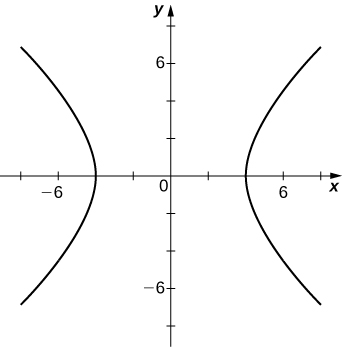36) $$\displaystyle x=8$$

For the following exercises, convert the rectangular equation to polar form and sketch its graph.

37) $$\displaystyle 3x−y=2$$

Solution: $$\displaystyle r=\frac{2}{3cosθ−sinθ}$$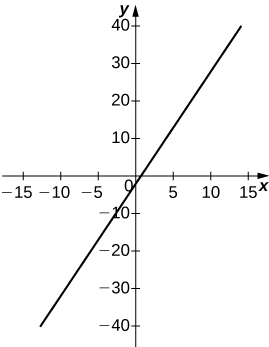38) $$\displaystyle y^2=4x$$

For the following exercises, convert the polar equation to rectangular form and sketch its graph.

39) $$\displaystyle r=4sinθ$$

40) $$\displaystyle x^2+y^2=4y$$

Solution:41) $$\displaystyle r=6cosθ$$

42) $$\displaystyle r=θ$$

Solution: $$\displaystyle xtan\sqrt{x^2+y^2}=y$$43) $$\displaystyle r=cotθcscθ$$

For the following exercises, sketch a graph of the polar equation and identify any symmetry.

44) $$\displaystyle r=1+sinθ$$

Solution: y-axis symmetry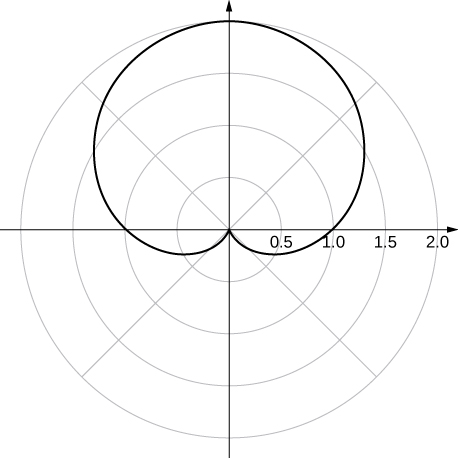45) $$\displaystyle r=3−2cosθ$$

46) $$\displaystyle r=2−2sinθ$$

Solution: y-axis symmetry47) $$\displaystyle r=5−4sinθ$$

48) $$\displaystyle r=3cos(2θ)$$

Solution: x- and y-axis symmetry and symmetry about the pole49) $$\displaystyle r=3sin(2θ)$$

50) $$\displaystyle r=2cos(3θ)$$

Solution: x-axis symmetry51) $$\displaystyle r=3cos(\frac{θ}{2})$$

52) $$\displaystyle r^2=4cos(\frac{2}{θ})$$

Solution: x- and y-axis symmetry and symmetry about the pole53) $$\displaystyle r^2=4sinθ$$

54) $$\displaystyle r=2θ$$

Solution: no symmetry55) [T] The graph of $$\displaystyle r=2cos(2θ)sec(θ).$$ is called a strophoid. Use a graphing utility to sketch the graph, and, from the graph, determine the asymptote.

56) [T] Use a graphing utility and sketch the graph of $$\displaystyle r=\frac{6}{2sinθ−3cosθ}$$.

Solution: a line57) [T] Use a graphing utility to graph $$\displaystyle r=\frac{1}{1−cosθ}$$.

58) [T] Use technology to graph $$\displaystyle r=e^{sin(θ)}−2cos(4θ)$$.

Solution:59) [T] Use technology to plot $$\displaystyle r=sin(\frac{3θ}{7})$$ (use the interval $$\displaystyle 0≤θ≤14π$$).

60) Without using technology, sketch the polar curve $$\displaystyle θ=\frac{2π}{3}$$.

Solution:61) [T] Use a graphing utility to plot $$\displaystyle r=θsinθ$$ for $$\displaystyle −π≤θ≤π$$.

62) [T] Use technology to plot $$\displaystyle r=e^{−0.1θ}$$ for $$\displaystyle −10≤θ≤10.$$

Solution:63) [T] There is a curve known as the “Black Hole.” Use technology to plot $$\displaystyle r=e^{−0.01θ}$$ for $$\displaystyle −100≤θ≤100$$.

64) [T] Use the results of the preceding two problems to explore the graphs of $$\displaystyle r=e^{−0.001θ}$$ and $$\displaystyle r=e^{−0.0001θ}$$ for $$\displaystyle |θ|>100$$.

Solution: Answers vary. One possibility is the spiral lines become closer together and the total number of spirals increases.

## 11.4: Area and Arc Length in Polar Coordinates

For the following exercises, determine a definite integral that represents the area.

1) Region enclosed by $$\displaystyle r=4$$

2) Region enclosed by $$\displaystyle r=3sinθ$$

Solution: $$\displaystyle \frac{9}{2}∫^π_0sin^2θdθ$$

3) Region in the first quadrant within the cardioid $$\displaystyle r=1+sinθ$$

4) Region enclosed by one petal of $$\displaystyle r=8sin(2θ)$$

Solution: $$\displaystyle \frac{3}{2}∫^{π/2}_0sin^2(2θ)dθ$$

5) Region enclosed by one petal of $$\displaystyle r=cos(3θ)$$

6) Region below the polar axis and enclosed by $$\displaystyle r=1−sinθ$$

Solution: $$\displaystyle \frac{1}{2}∫^{2π}_π(1−sinθ)^2dθ$$

7) Region in the first quadrant enclosed by $$\displaystyle r=2−cosθ$$

8) Region enclosed by the inner loop of $$\displaystyle r=2−3sinθ$$

Solution: $$\displaystyle ∫^{π/2}_{sin^{−1}(2/3)}(2−3sinθ)^2dθ$$

9) Region enclosed by the inner loop of $$\displaystyle r=3−4cosθ$$

10) Region enclosed by $$\displaystyle r=1−2cosθ$$ and outside the inner loop

Solution: $$\displaystyle ∫^π_0(1−2cosθ)^2dθ−∫^{π/3}_0(1−2cosθ)^2dθ$$

11) Region common to $$\displaystyle r=3sinθ$$ and $$\displaystyle r=2−sinθ$$

12) Region common to $$\displaystyle r=2$$ and $$\displaystyle r=4cosθ$$

Solution: $$\displaystyle 4∫^{π/3}_0dθ+16∫^{π/2}_{π/3}(cos^2θ)dθ$$

13) Region common to $$\displaystyle r=3cosθ$$ and $$\displaystyle r=3sinθ$$

For the following exercises, find the area of the described region.

14) Enclosed by $$\displaystyle r=6sinθ$$

Solution: $$\displaystyle 9π$$

15) Above the polar axis enclosed by $$\displaystyle r=2+sinθ$$

16) Below the polar axis and enclosed by $$\displaystyle r=2−cosθ$$

Solution: $$\displaystyle \frac{9π}{4}$$

17) Enclosed by one petal of $$\displaystyle r=4cos(3θ)$$

18) Enclosed by one petal of $$\displaystyle r=3cos(2θ)$$

Solution: $$\displaystyle \frac{9π}{8}$$

19) Enclosed by $$\displaystyle r=1+sinθ$$

20) Enclosed by the inner loop of $$\displaystyle r=3+6cosθ$$

Solution: $$\displaystyle \frac{18π−27\sqrt{3}}{2}$$

21) Enclosed by $$\displaystyle r=2+4cosθ$$ and outside the inner loop

22) Common interior of $$\displaystyle r=4sin(2θ)$$ and $$\displaystyle r=2$$

Solution: $$\displaystyle \frac{4}{3}(4π−3\sqrt{3})$$

23) Common interior of $$\displaystyle r=3−2sinθ$$ and $$\displaystyle r=−3+2sinθ$$

24) Common interior of $$\displaystyle r=6sinθ$$ and $$\displaystyle r=3$$

Solution: $$\displaystyle 32(4π−33√) 25) Inside \(\displaystyle r=1+cosθ$$ and outside $$\displaystyle r=cosθ$$

26) Common interior of $$\displaystyle r=2+2cosθ$$ and $$\displaystyle r=2sinθ$$

Solution: $$\displaystyle 2π−4$$

For the following exercises, find a definite integral that represents the arc length.

27) $$\displaystyle r=4cosθ$$ on the interval $$\displaystyle 0≤θ≤\frac{π}{2}$$

28) $$\displaystyle r=1+sinθ$$ on the interval $$\displaystyle 0≤θ≤2π$$

Solution: $$\displaystyle ∫^{2π}_0\sqrt{(1+sinθ)^2+cos^2θ}dθ$$

29) $$\displaystyle r=2secθ$$ on the interval $$\displaystyle 0≤θ≤\frac{π}{3}$$

30) $$\displaystyle r=e^θ$$ on the interval $$\displaystyle 0≤θ≤1$$

Solution: $$\displaystyle \sqrt{2}∫^1_0e^θdθ$$

For the following exercises, find the length of the curve over the given interval.

31) $$\displaystyle r=6$$ on the interval $$\displaystyle 0≤θ≤\frac{π}{2}$$

32) $$\displaystyle r=e^{3θ}$$ on the interval $$\displaystyle 0≤θ≤2$$

Solution: $$\displaystyle \frac{\sqrt{10}}{3}(e^6−1)$$

33) $$\displaystyle r=6cosθ$$ on the interval $$\displaystyle 0≤θ≤\frac{π}{2}$$

34) $$\displaystyle r=8+8cosθ$$ on the interval $$\displaystyle 0≤θ≤π$$

Solution: 32

35) $$\displaystyle r=1−sinθ$$ on the interval $$\displaystyle 0≤θ≤2π$$

For the following exercises, use the integration capabilities of a calculator to approximate the length of the curve.

36) [T] $$\displaystyle r=3θ$$ on the interval $$\displaystyle 0≤θ≤\frac{π}{2}$$

Solution: 6.238

37) [T] $$\displaystyle r=\frac{2}{θ}$$ on the interval $$\displaystyle π≤θ≤2π$$

38) [T] $$\displaystyle r=sin^2(\frac{θ}{2})$$ on the interval $$\displaystyle 0≤θ≤π$$

Solution: 2

39) [T] $$\displaystyle r=2θ^2$$ on the interval $$\displaystyle 0≤θ≤π$$

40) [T] $$\displaystyle r=sin(3cosθ)$$ on the interval $$\displaystyle 0≤θ≤π$$

Solution: 4.39

For the following exercises, use the familiar formula from geometry to find the area of the region described and then confirm by using the definite integral.

41) $$\displaystyle r=3sinθ$$ on the interval $$\displaystyle 0≤θ≤π$$

42) $$\displaystyle r=sinθ+cosθ$$ on the interval $$\displaystyle 0≤θ≤π$$

Solution: $$\displaystyle A=π(\frac{\sqrt{2}}{2})^2=\frac{π}{2}$$ and $$\displaystyle \frac{1}{2}∫^π_0(1+2sinθcosθ)dθ=\frac{π}{2}$$

43) $$\displaystyle r=6sinθ+8cosθ$$ on the interval $$\displaystyle 0≤θ≤π$$

For the following exercises, use the familiar formula from geometry to find the length of the curve and then confirm using the definite integral.

44) $$\displaystyle r=3sinθ$$ on the interval $$\displaystyle 0≤θ≤π$$

Solution: $$\displaystyle C=2π(\frac{3}{2})=3π$$ and $$\displaystyle ∫^π_03dθ=3π$$

45) $$\displaystyle r=sinθ+cosθ$$ on the interval $$\displaystyle 0≤θ≤π$$

46) $$\displaystyle r=6sinθ+8cosθ$$ on the interval $$\displaystyle 0≤θ≤π$$

Solution: $$\displaystyle C=2π(5)=10π$$ and $$\displaystyle ∫^π_010dθ=10π$$

47) Verify that if $$\displaystyle y=rsinθ=f(θ)sinθ$$ then $$\displaystyle \frac{dy}{dθ}=f'(θ)sinθ+f(θ)cosθ.$$

For the following exercises, find the slope of a tangent line to a polar curve $$\displaystyle r=f(θ)$$. Let $$\displaystyle x=rcosθ=f(θ)cosθ$$ and $$\displaystyle y=rsinθ=f(θ)sinθ$$, so the polar equation $$\displaystyle r=f(θ)$$ is now written in parametric form.

48) Use the definition of the derivative $$\displaystyle dydx=\frac{dy/dθ}{dx/dθ}$$ and the product rule to derive the derivative of a polar equation.

Solution: $$\displaystyle \frac{dy}{dx}=\frac{f′(θ)sinθ+f(θ)cosθ}{f′(θ)cosθ−f(θ)sinθ}$$

49) $$\displaystyle r=1−sinθ; (\frac{1}{2},\frac{π}{6})$$

50) $$\displaystyle r=4cosθ; (2,\frac{π}{3})$$

Solution: The slope is $$\displaystyle \frac{1}{\sqrt{3}}$$.

51) $$\displaystyle r=8sinθ; (4,\frac{5π}{6})$$

52) $$\displaystyle r=4+sinθ; (3,\frac{3π}{2})$$

Solution: The slope is 0.

53) $$\displaystyle r=6+3cosθ; (3,π)$$

54) $$\displaystyle r=4cos(2θ);$$ tips of the leaves

Solution: At $$\displaystyle (4,0),$$ the slope is undefined. At $$\displaystyle (−4,\frac{π}{2})$$, the slope is 0.

55) $$\displaystyle r=2sin(3θ);$$ tips of the leaves

56) $$\displaystyle r=2θ; (\frac{π}{2},\frac{π}{4})$$

Solution: The slope is undefined at $$\displaystyle θ=\frac{π}{4}$$.

57) Find the points on the interval $$\displaystyle −π≤θ≤π$$ at which the cardioid $$\displaystyle r=1−cosθ$$ has a vertical or horizontal tangent line.

58) For the cardioid $$\displaystyle r=1+sinθ,$$ find the slope of the tangent line when $$\displaystyle θ=\frac{π}{3}$$.

Solution: Slope = −1.

For the following exercises, find the slope of the tangent line to the given polar curve at the point given by the value of $$\displaystyle θ$$.

59) $$\displaystyle r=3cosθ,θ=\frac{π}{3}$$

60) $$\displaystyle r=θ, θ=\frac{π}{2}$$

Soltuion: Slope is $$\displaystyle \frac{−2}{π}$$.

61) $$\displaystyle r=lnθ, θ=e$$

62) [T] Use technology: $$\displaystyle r=2+4cosθ$$ at $$\displaystyle θ=\frac{π}{6}$$

Solution: Calculator answer: −0.836.

For the following exercises, find the points at which the following polar curves have a horizontal or vertical tangent line.

63) $$\displaystyle r=4cosθ$$

64) $$\displaystyle r^2=4cos(2θ)$$

Solution: Horizontal tangent at $$\displaystyle (±\sqrt{2},\frac{π}{6}), (±\sqrt{2},−\frac{π}{6})$$.

65) $$\displaystyle r=2sin(2θ)$$

66) The cardioid $$\displaystyle r=1+sinθ$$

Solution: Horizontal tangents at $$\displaystyle \frac{π}{2},\frac{7π}{6},\frac{11π}{6}.$$ Vertical tangents at $$\displaystyle \frac{π}{6},\frac{5π}{6}$$ and also at the pole $$\displaystyle (0,0)$$.

67) Show that the curve $$\displaystyle r=sinθtanθ$$ (called a cissoid of Diocles) has the line $$\displaystyle x=1$$ as a vertical asymptote.

## 11.5: Conic Sections

For the following exercises, determine the equation of the parabola using the information given.

1) Focus $$\displaystyle (4,0)$$ and directrix $$\displaystyle x=−4$$

Solution: $$\displaystyle y^2=16x$$

2) Focus $$\displaystyle (0,−3)$$ and directrix $$\displaystyle y=3$$

3) Focus $$\displaystyle (0,0.5)$$ and directrix $$\displaystyle y=−0.5$$

Solution: $$\displaystyle x^2=2y$$

4) Focus $$\displaystyle (2,3)$$ and directrix $$\displaystyle x=−2$$

5) Focus $$\displaystyle (0,2)$$ and directrix $$\displaystyle y=4$$

Solution: $$\displaystyle x^2=−4(y−3)$$

6) Focus $$\displaystyle (−1,4)$$ and directrix $$\displaystyle x=5$$

7) Focus $$\displaystyle (−3,5)$$ and directrix $$\displaystyle y=1$$

Solution: $$\displaystyle (x+3)^2=8(y−3)$$

8) Focus $$\displaystyle (\frac{5}{2},−4)$$ and directrix $$\displaystyle x=\frac{7}{2}$$

For the following exercises, determine the equation of the ellipse using the information given.

9) Endpoints of major axis at $$\displaystyle (4,0),(−4,0)$$ and foci located at $$\displaystyle (2,0),(−2,0)$$

Solution: $$\displaystyle \frac{x^2}{16}+\frac{y^2}{12}=1$$

10) Endpoints of major axis at $$\displaystyle (0,5),(0,−5)$$ and foci located at $$\displaystyle (0,3),(0,−3)$$

11) Endpoints of major axis at $$\displaystyle (0,2),(0,−2)$$ and foci located at $$\displaystyle (3,0),(−3,0)$$

Solution: $$\displaystyle \frac{x^2}{13}+\frac{y^2}{4}=1$$

12) Endpoints of major axis at $$\displaystyle (−3,3),(7,3)$$ and foci located at $$\displaystyle (−2,3),(6,3)$$

13) Endpoints of major axis at $$\displaystyle (−3,5),(−3,−3)$$ and foci located at $$\displaystyle (−3,3),(−3,−1)$$

Solution: $$\displaystyle \frac{(y−1)^2}{16}+\frac{(x+3)^2}{12}=1$$

14) Endpoints of major axis at $$\displaystyle (0,0),(0,4)$$ and foci located at $$\displaystyle (5,2),(−5,2)$$

15) Foci located at $$\displaystyle (2,0),(−2,0)$$ and eccentricity of $$\displaystyle \frac{1}{2}$$

Solutin: $$\displaystyle \frac{x^2}{16}+\frac{y^2}{12}=1$$

16) Foci located at $$\displaystyle (0,−3),(0,3)$$ and eccentricity of $$\displaystyle \frac{3}{4}$$

For the following exercises, determine the equation of the hyperbola using the information given.

17) Vertices located at $$\displaystyle (5,0),(−5,0)$$ and foci located at $$\displaystyle (6,0),(−6,0)$$

Solution: $$\displaystyle \frac{x^2}{25}−\frac{y^2}{11}=1$$

18) Vertices located at $$\displaystyle (0,2),(0,−2)$$ and foci located at $$\displaystyle (0,3),(0,−3)$$

19) Endpoints of the conjugate axis located at $$\displaystyle (0,3),(0,−3)$$ and foci located $$\displaystyle (4,0),(−4,0)$$

Solution: $$\displaystyle \frac{x^2}{7}−\frac{y^2}{9}=1$$

20) Vertices located at $$\displaystyle (0,1),(6,1)$$ and focus located at $$\displaystyle (8,1)$$

21) Vertices located at $$\displaystyle (−2,0),(−2,−4)$$ and focus located at $$\displaystyle (−2,−8)$$

Solution: $$\displaystyle \frac{(y+2)^2}{4}−\frac{(x+2)^2}{32}=1$$

22) Endpoints of the conjugate axis located at $$\displaystyle (3,2),(3,4)$$ and focus located at $$\displaystyle (3,7)$$

23) Foci located at $$\displaystyle (6,−0),(6,0)$$ and eccentricity of $$\displaystyle 3$$

Soluton: $$\displaystyle \frac{x^2}{4}−\frac{y^2}{32}=1$$

24) $$\displaystyle (0,10),(0,−10)$$ and eccentricity of 2.5

For the following exercises, consider the following polar equations of conics. Determine the eccentricity and identify the conic.

25) $$\displaystyle r=\frac{−1}{1+cosθ}$$

Solution: $$\displaystyle e=1,$$ parabola

26) $$\displaystyle r=\frac{8}{2−sinθ}$$

27) $$\displaystyle r=\frac{5}{2+sinθ}$$

Solution: $$\displaystyle e=\frac{1}{2},$$ ellipse

28) $$\displaystyle r=\frac{5}{−1+2sinθ}$$

29) $$\displaystyle r=\frac{3}{2−6sinθ}$$

Solution: $$\displaystyle e=3$$, hyperbola

30) $$\displaystyle r=\frac{3}{−4+3sinθ}$$

For the following exercises, find a polar equation of the conic with focus at the origin and eccentricity and directrix as given.

31) Directrix: $$\displaystyle x=4;e=\frac{1}{5}$$

Solution: $$\displaystyle r=\frac{4}{5+cosθ}$$

32) Directrix: $$\displaystyle x=−4;e=5$$

33) Directrix: $$\displaystyle y=2;e=2$$

Solution: $$\displaystyle r=\frac{4}{1+2sinθ}$$

34) Directrix: $$\displaystyle y=−2;e=\frac{1}{2}$$

For the following exercises, sketch the graph of each conic.

35) $$\displaystyle r=\frac{1}{1+sinθ}$$

Solution:36) $$\displaystyle r=\frac{1}{1−cosθ}$$

37) $$\displaystyle r=\frac{4}{1+cosθ}$$

Solution:38) $$\displaystyle r=\frac{10}{5+4sinθ}$$

39) $$\displaystyle r=\frac{15}{3−2cosθ}$$

Soluton:40) $$\displaystyle r=\frac{32}{3+5sinθ}$$

41) $$\displaystyle r(2+sinθ)=4$$

Solution:42) $$\displaystyle r=\frac{3}{2+6sinθ}$$

43) $$\displaystyle r=\frac{3}{−4+2sinθ}$$

Solution:44) $$\displaystyle \frac{x^2}{9}+\frac{y^2}{4}=1$$

45) $$\displaystyle \frac{x^2}{4}+\frac{y^2}{16}=1$$

Solution:46) $$\displaystyle 4x^2+9y^2=36$$

47) $$\displaystyle 25x^2−4y^2=100$$

Solution:48) $$\displaystyle \frac{x^2}{16}−\frac{y^2}{9}=1$$

49) $$\displaystyle x^2=12y$$

Solution: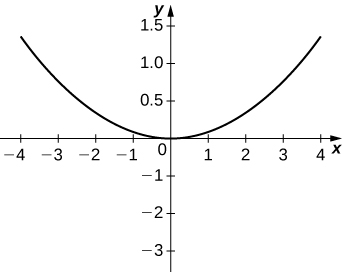50) $$\displaystyle y^2=20x$$

51) $$\displaystyle 12x=5y^2$$

Solution:For the following equations, determine which of the conic sections is described.

52) $$\displaystyle xy=4$$

53) $$\displaystyle x^2+4xy−2y^2−6=0$$

Solution: Hyperbola

54) $$\displaystyle x^2+2\sqrt{3}xy+3y^2−6=0$$

55) $$\displaystyle x^2−xy+y^2−2=0$$

Solution: Ellipse

56) $$\displaystyle 34x^2−24xy+41y^2−25=0$$

57) $$\displaystyle 52x^2−72xy+73y^2+40x+30y−75=0$$

Solution: Ellipse

58) The mirror in an automobile headlight has a parabolic cross section, with the lightbulb at the focus. On a schematic, the equation of the parabola is given as $$\displaystyle x^2=4y$$. At what coordinates should you place the lightbulb?

59) A satellite dish is shaped like a paraboloid of revolution. The receiver is to be located at the focus. If the dish is 12 feet across at its opening and 4 feet deep at its center, where should the receiver be placed?

Solution: At the point 2.25 feet above the vertex.

60) Consider the satellite dish of the preceding problem. If the dish is 8 feet across at the opening and 2 feet deep, where should we place the receiver?

61) A searchlight is shaped like a paraboloid of revolution. A light source is located 1 foot from the base along the axis of symmetry. If the opening of the searchlight is 3 feet across, find the depth.

Solution: 0.5625 feet

62) Whispering galleries are rooms designed with elliptical ceilings. A person standing at one focus can whisper and be heard by a person standing at the other focus because all the sound waves that reach the ceiling are reflected to the other person. If a whispering gallery has a length of 120 feet and the foci are located 30 feet from the center, find the height of the ceiling at the center.

63) A person is standing 8 feet from the nearest wall in a whispering gallery. If that person is at one focus and the other focus is 80 feet away, what is the length and the height at the center of the gallery?

Solution: Length is 96 feet and height is approximately 26.53 feet.

For the following exercises, determine the polar equation form of the orbit given the length of the major axis and eccentricity for the orbits of the comets or planets. Distance is given in astronomical units (AU).

64) Halley’s Comet: length of major axis = 35.88, eccentricity = 0.967

65) Hale-Bopp Comet: length of major axis = 525.91, eccentricity = 0.995

Solution: $$\displaystyle r=\frac{2.616}{1+0.995cosθ}$$

66) Mars: length of major axis = 3.049, eccentricity = 0.0934

67) Jupiter: length of major axis = 10.408, eccentricity = 0.0484

Solution: $$\displaystyle r=\frac{5.192}{1+0.0484cosθ}$$

## Chapter Review Exercises

True or False? Justify your answer with a proof or a counterexample.

1) The rectangular coordinates of the point $$\displaystyle (4,\frac{5π}{6})$$ are $$\displaystyle (2\sqrt{3},−2).$$

2) The equations $$\displaystyle x=cosh(3t), y=2sinh(3t)$$ represent a hyperbola.

Solution: True.

3) The arc length of the spiral given by $$\displaystyle r=\frac{θ}{2}$$ for $$\displaystyle 0≤θ≤3π$$ is $$\displaystyle \frac{9}{4}π^3$$.

4) Given $$\displaystyle x=f(t)$$ and $$\displaystyle y=g(t)$$, if $$\displaystyle \frac{dx}{dy}=\frac{dy}{dx}$$, then $$\displaystyle f(t)=g(t)+C,$$ where $$\displaystyle C$$ is a constant.

Solution: False. Imagine $$\displaystyle y=t+1, x=−t+1.$$

For the following exercises, sketch the parametric curve and eliminate the parameter to find the Cartesian equation of the curve.

5) $$\displaystyle x=1+t, y=t^2−1, −1≤t≤1$$

6) $$\displaystyle x=e^t, y=1−e^{3t}, 0≤t≤1$$

Solution: $$\displaystyle y=1−x^3$$7) $$\displaystyle x=sinθ, y=1−cscθ, 0≤θ≤2π$$

8) $$\displaystyle x=4cosϕ, y=1−sinϕ, 0≤ϕ≤2π$$

Solution: $$\displaystyle \frac{x^2}{16}+(y−1)^2=1$$For the following exercises, sketch the polar curve and determine what type of symmetry exists, if any.

9) $$\displaystyle r=4sin(\frac{θ}{3})$$

10) $$\displaystyle r=5cos(5θ)$$

Solution: Symmetric about polar axisFor the following exercises, find the polar equation for the curve given as a Cartesian equation.

11) $$\displaystyle x+y=5$$

12) $$\displaystyle y^2=4+x^2$$

Solution: $$\displaystyle r^2=\frac{4}{sin^2θ−cos^2θ}$$

For the following exercises, find the equation of the tangent line to the given curve. Graph both the function and its tangent line.

13) $$\displaystyle x=ln(t), y=t^2−1, t=1$$

14) $$\displaystyle r=3+cos(2θ), θ=\frac{3π}{4}$$

Solution: $$\displaystyle y=\frac{3\sqrt{2}}{2}+\frac{1}{5}(x+\frac{3\sqrt{2}}{2})$$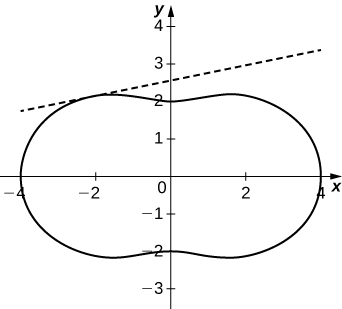15) Find $$\displaystyle \frac{dy}{dx}, \frac{dx}{dy},$$ and $$\displaystyle \frac{d^2x}{dy^2}$$ of $$\displaystyle y=(2+e^{−t}), x=1−sin(t)$$

For the following exercises, find the area of the region.

16) $$\displaystyle x=t^2, y=ln(t), 0≤t≤e$$

Solution: $$\displaystyle \frac{e^2}{2}$$

17) $$\displaystyle r=1−sinθ$$ in the first quadrant

For the following exercises, find the arc length of the curve over the given interval.

18) $$\displaystyle x=3t+4, y=9t−2, 0≤t≤3$$

Solution: $$\displaystyle 9\sqrt{10}$$

19) $$\displaystyle r=6cosθ, 0≤θ≤2π.$$ Check your answer by geometry.

For the following exercises, find the Cartesian equation describing the given shapes.

20) A parabola with focus $$\displaystyle (2,−5)$$ and directrix $$\displaystyle x=6$$

Solution: $$\displaystyle (y+5)^2=−8x+32$$

21) An ellipse with a major axis length of 10 and foci at $$\displaystyle (−7,2)$$ and $$\displaystyle (1,2)$$

22) A hyperbola with vertices at $$\displaystyle (3,−2)$$ and $$\displaystyle (−5,−2)$$ and foci at $$\displaystyle (−2,−6)$$ and $$\displaystyle (−2,4)$$

Solution: $$\displaystyle \frac{(y+1)^2}{16}−\frac{(x+2)^2}{9}=1$$

For the following exercises, determine the eccentricity and identify the conic. Sketch the conic.

23) $$\displaystyle r=\frac{6}{1+3cos(θ)}$$

24) $$\displaystyle r=\frac{4}{3−2cosθ}$$

Solution: $$\displaystyle e=\frac{2}{3}$$, ellipse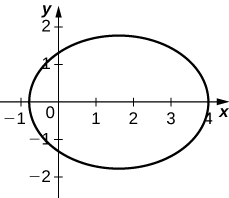25) $$\displaystyle r=\frac{7}{5−5cosθ}$$

26) Determine the Cartesian equation describing the orbit of Pluto, the most eccentric orbit around the Sun. The length of the major axis is 39.26 AU and minor axis is 38.07 AU. What is the eccentricity?

Solution: $$\displaystyle \frac{y^2}{19.03^2}+\frac{x^2}{19.63^2}=1, e=0.2447$$

27) The C/1980 E1 comet was observed in 1980. Given an eccentricity of 1.057 and a perihelion (point of closest approach to the Sun) of 3.364 AU, find the Cartesian equations describing the comet’s trajectory. Are we guaranteed to see this comet again? (Hint: Consider the Sun at point $$\displaystyle (0,0)$$.)